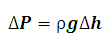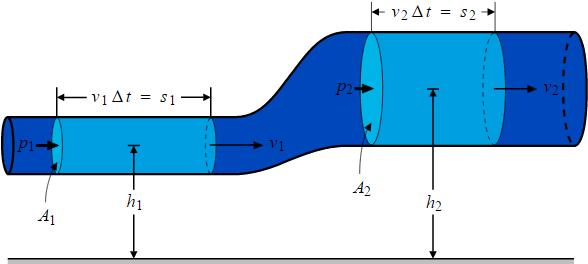# Pascal's Law Calculator

This Calctown Calculator calculates the change in fluid pressure with change in altitude.

kg/m3
m

#### Result

N/m2where

P1 and P2 are respective fluid pressures at heights h1 and h2

g = acceleration due to gravity

ρ = fluid density

ΔP = P2-P1 = ρg(h2-h1) = ρgΔh